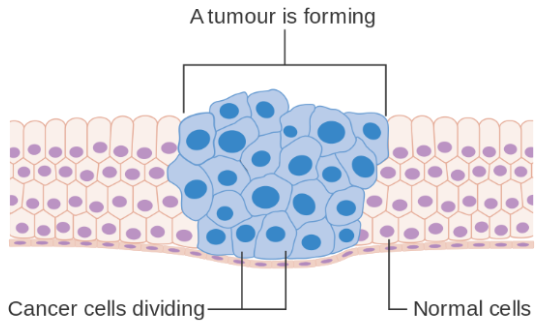# Applications of Calculus: Modeling Tumor GrowthCalculus can help us model the growth of tumors.

Tumors appear to grow exponentially early in their lifecycles, which means that if a tumor’s volume at time $t$ is given by $V(t)$ and its growth rate is a constant $r,$ then

\begin{align*} \frac{dV}{dt} = rV. \end{align*}

However, tumors do not grow exponentially forever: it has been observed that after some time, tumor growth slows down.

To incorporate this into our model, instead of setting the growth rate to a constant r, we can set it to an exponential decay function given by

\begin{align*} r(t) = r_0e^{-kt}. \end{align*}

Then the full model is

\begin{align*} \frac{dV}{dt} = r_0e^{-kt}V. \end{align*}

We can separate variables and integrate to solve for $V:$

\begin{align*} \frac{dV}{dt} &= r_0e^{-kt}V \\[5pt] \frac{dV}{V} &= r_0e^{-kt}dt \\[5pt] \int \frac{dV}{V} &= \int r_0e^{-kt}dt \\[5pt] \ln V &= \mbox{constant} + \int r_0e^{-kt}dt \\[5pt] V &= e^{\mbox{constant} + \int r_0e^{-kt}dt} \\[5pt] V &= (\mbox{constant})e^{\int r_0e^{-kt}dt} \\[5pt] V &= (\mbox{constant})e^{\mbox{constant} - \frac{r_0}{k}e^{-kt}} \\[5pt] V &= C_1e^{C_2 - \frac{r_0}{k}e^{-kt}} \end{align*}

We can solve for one of the constants in terms of the initial volume and the other constant:

\begin{align*} V_0 &= V(t=0) \\[5pt] V_0 &= C_1e^{C_2 - \frac{r_0}{k}} \\[5pt] C_1 &= V_0e^{\frac{r_0}{k} - C_2} \end{align*}

When we plug the constant back in, it cancels out the other constant to yield a final formula:

\begin{align*} V &= \left( V_0e^{\frac{r_0}{k}-C_2} \right) e^{C_2-\frac{r_0}{k}e^{-kt}} \\[5pt] &= V_0 e^{\frac{r_0}{k} - C_2 + C_2 - \frac{r_0}{k}e^{-kt}} \\[5pt] &= V_0 e^{\frac{r_0}{k}\left(1-e^{-kt} \right)} \end{align*}

This is called the Gompertz function, and it has been used to model tumor growth and measure the effectiveness of tumor-killing treatments.

Tags: